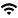# Computer Science Speaker Series Master Calendar

View Full Calendar

## Theory Seminar: Young-San- Online Directed Spanners and Steiner Forests

Event Type
Seminar/Symposium
Chandra Chekuri
VirtualDate
Oct 4, 2021   10:00 am
Views
33
Originating Calendar
Computer Science Speakers Calendar

The zoom details are:

Meeting ID: 852 3676 8481

The details of the talk are:

Title: Online Directed Spanners and Steiner Forests

Abstract: We present online algorithms for directed spanners and directed Steiner forests. These are well-studied network connectivity problems that fall under the unifying framework of online covering and packing linear programming formulations. This framework was developed in the seminal work of Buchbinder and Naor (Mathematics of Operations Research, 34, 2009) and is based on primal-dual techniques.
Specifically, our results include the following:

1. For the pairwise spanner problem, in which the pairs of vertices to be spanned arrive online, we present an efficient randomized algorithm with competitive ratio $\tilde{O}(n^{4/5})$ for graphs with general lengths, where $n$ is the number of vertices of the given graph. For graphs with uniform lengths, we give an efficient randomized algorithm with competitive ratio $\tilde{O}(n^{2/3+\epsilon})$, and an efficient deterministic algorithm with competitive ratio $\tilde{O}(k^{1/2+\epsilon})$, where $k$ is the number of terminal pairs. To the best of our knowledge, these are the first online algorithms for directed spanners.
In the offline version, the current best approximation ratio for uniform edge lengths is $\tilde{O}(n^{3/5 + \epsilon})$, due to Chlamtac, Dinitz, Kortsarz, and Laekhanukit (SODA 2017, TALG 2020).

2. For the directed Steiner forest problem with uniform costs, in which the pairs of vertices to be connected arrive online, we present an efficient randomized algorithm with competitive ratio $\tilde{O}(n^{2/3 + \epsilon})$. The state-of-the-art online algorithm for general costs is due to Chakrabarty, Ene, Krishnaswamy, and Panigrahi (SICOMP 2018) and is $\tilde{O}(k^{1/2 + \epsilon})$-competitive. In the offline version, the current best approximation ratio with uniform costs is $\tilde{O}(n^{26/45 + \epsilon})$, due to Abboud and Bodwin (SODA 2018).

To obtain efficient and competitive online algorithms, we observe that a small modification of the online covering and packing framework by Buchbinder and Naor implies a polynomial-time implementation of the primal-dual approach with separation oracles, which a priori might perform exponentially many calls to the oracle. We convert the online spanner problem into an online covering problem and complete the rounding-step analysis in a problem-specific fashion.

This is joint work with Elena Grigorescu and Kent Quanrud